Data Structures and Algorithms with Object-Oriented Design Patterns in C++## Application: Typesetting Problem

Consider the problem of typesetting a paragraph of justified text. A paragraph can be viewed as a sequence of n>0 words,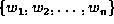. The objective is to determine how to break the sequence into individual lines of text of the appropriate size. Each word is separated from the next by some amount of space. By stretching or compressing the space between the words, the left and right ends of consecutive lines of text are made to line up. A paragraph looks best when the amount of stretching or compressing is minimized.

We can formulate the problem as follows: Assume that we are given the lengths of the words,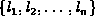, and that the desired length of a line is D. Let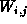represent the sequence of words from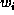to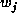(inclusive). I.e.,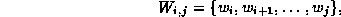for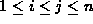.

Let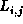be the sum of the lengths of the words in the sequence. I.e.,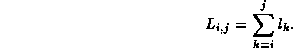The natural length, for the sequenceis the sum of the lengths of the words,plus the normal amount of space between those words. Let s be the normal size of the space between two words. Then the natural length ofis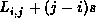. Note, we can also definerecursively as follows: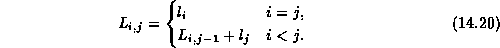In general, when we typeset the sequenceall on a single line, we need to stretch or compress the spaces between the words so that the length of the line is the desired length D. Therefore, the amount of stretching or compressing is given by the difference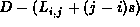. However, if the sum of the lengths of the words,, is longer than the desired line length D, it is not possible to typeset the sequence on a single line.

Let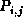be the penalty associated with typesetting the sequenceon a single line. Then,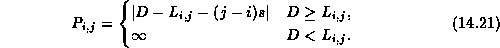This definition is of penalty is consistent with the stated objectives: The penalty increases as the difference between the natural length of the sequence and the desired length increases and the infinite penalty disallows lines that are too long.

Finally, we define the quantity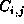foras the minimum total penalty required to typeset the sequence. In this case, the text may be all on one line or it may be split over more than one line. The quantityis given by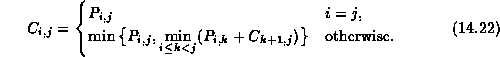We obtain Equationas follows: When i=j there is only one word in the paragraph. The minimum total penalty associated with typesetting the paragraph in this case is just the penalty which results from putting the one word on a single line.

In the general case, there is more than one word in the sequence. In order to determine the optimal way in which to typeset the paragraph we consider the cost of putting the first k words of the sequence on the first line of the paragraph,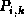, plus the minimum total cost associated with typesetting the rest of the paragraph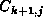. The value of k which minimizes the total cost also specifies where the line break should occur.Copyright © 1997 by Bruno R. Preiss, P.Eng. All rights reserved.